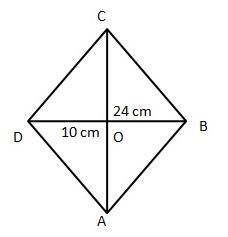# Test: Types And Angle Sum Property

## 10 Questions MCQ Test Mathematics (Maths) Class 9 | Test: Types And Angle Sum Property

Description
This mock test of Test: Types And Angle Sum Property for Class 9 helps you for every Class 9 entrance exam. This contains 10 Multiple Choice Questions for Class 9 Test: Types And Angle Sum Property (mcq) to study with solutions a complete question bank. The solved questions answers in this Test: Types And Angle Sum Property quiz give you a good mix of easy questions and tough questions. Class 9 students definitely take this Test: Types And Angle Sum Property exercise for a better result in the exam. You can find other Test: Types And Angle Sum Property extra questions, long questions & short questions for Class 9 on EduRev as well by searching above.
QUESTION: 1

Solution:
QUESTION: 2

### The opposite angles of a parallelogram are (3x – 2)° and (50 – x)° the measure of these angles is

Solution:

The opposite angles in a parallelogram are equal.

So,1st angle=2nd anlge

• 1st angle = 3x-2
• 2nd angle = 50-x

so, equate both the angles

3x-2=50-x

3x+x=50+2

4x=52

x=52/4

x=13

now, substitute the value of x

therefore,

1st angle = 3x-2

1st angle = 39-2 = 37o

2nd angle = 50-x

2nd angle = 50-13 = 37o

QUESTION: 3

### The diagonals of a rhombus are 10 cm and 24 cm. find the length of a side of the rhombus.

Solution:We know that in a rhombus the diagonals bisect each other at 90∘90∘

AC=24cm

BD=10cm

BO=5cm

=122+52

=144+25

=169

AB=√169

=13cm

Hence the length of the rhombus is 13 cm

QUESTION: 4

In quadrilateral PQRS, if ∠P = 60° and ∠Q : ∠R : ∠S = 2 : 3 : 7, then ∠S =

Solution:

Let ∠Q = 2x, ∠R = 3x and ∠S = 7x

Now, ∠P + ∠Q + ∠R + ∠S = 360°

⇒ 60° + 2x + 3x + 7x = 360°

⇒ 12x = 300°

⇒ x =  300°/ 12 = 25°

∠S = 7x = 7 × 25° = 175°

QUESTION: 5

Which of the following is not a parallelogram?

Solution:

Both trapezium and parallelogram are quadrilaterals. A parallelogram has two pairs of parallel sides. A trapezoid only has one pair of parallel sides.

QUESTION: 6

The angles of a quadrilateral are (5x)°, (3x + 10)°, (6x – 20)° and (x + 25)°. Now, the measure of each angle of the quadrilateral will be

Solution: Sum of all angle of quadrilateral=360dgree
5x+3x+10+6x-20+x+25=360
=15x+15=360
=15x=360-15
=15x=345
=x=23
First angle=5x=5×23=115
Second angle=3x+10=3×23+10=79
Third angle=6x-20=6×23-20=118
Fourth angle=x+25=23+25=48.(Ans).
QUESTION: 7

The angles of a quadrilateral are in the ratio 3:5:9:13. The measure of angles of quadrilateral are:

Solution:

Let the angles be 3x, 5x, 9x, 13x

3x + 5x + 9x + 12x = 360o.

30x = 360o

x = 120

Angles are 36o, 60o, 108o, 156o.

QUESTION: 8

Two angles of a quadrilateral are 50° and 80° and other two angles are in the ratio 8 : 15. Find the measure of the remaining two angles.​

Solution: Let the other two angles be 8x and 15x
{by angle sum property of a quadrilateral=360०}

solution= 80०+50०+8x+15x=360०
130०+23x=360०
23x =360०-130०
=230०
23x=230०
x=230÷23
x=10
putting the value of x =10
8x = 8×10=80
15x=15×10=150
so other two angles which is in ratio 8:15 is
8o० and 150०
QUESTION: 9

All the angles of a convex quadrilateral are congruent. However, not all its sides are congruent. What type of quadrilateral is it?

Solution:

All the angles of a convex quadrilateral are congruent .

∴ Each angle = 900

Thus, the quadrilateral can be either square or rectangle.

Since all the sides are not equal, the quadrilateral is a rectangle because in rectangle only opposite sides are equal.

QUESTION: 10

The type of polygon in which its all angles and sides are equal is classified as

Solution: A polygon in which all sides are equal is called equilateral polygon and a polygon in which all angles are equal is called equiangular polygon polygon in which all sides and all angles are equal is called a regular polygon so the answer will be regular polygon